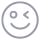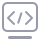mysql中sql语句的妙用

3 篇文章 0 订阅

1.在项目的实际开发过程中，我们经常会遇到这样的情况（例：我们需要某个时间段用户名字，用户发布的有效需求数，用户需求中标数，用户发布产品数，用户购买产品数；而我们现在有的表有（仅列出有效字段）：用户信息表（用户id，用户名字），需求表（用户id，需求id，需求审核状态），需求中标表（用户id，中标状态），产品表（用户id，产品id，产品审核状态），订单表（用户id，订单编号））

SELECT user_id, user, sum( need_count ) , sum( t_count ),sum(goods_count), (sum( need_count )+sum( t_count )+sum(goods_count)) total_num, sum(data)
FROM (
(

SELECT user_id, count( id ) AS need_count, 0 AS t_count , 0 AS goods_count , 0 AS data
WHERE examine =1 and time > 1493136000 and time < 1495814400
GROUP BY user_id
)
UNION ALL (

SELECT t_user_id AS user_id, 0 AS need_count, count( id ) AS t_count , 0 AS goods_count , 0 AS data
WHERE state =1 and winning_time > 1493136000 and winning_time < 1495814400
GROUP BY t_user_id
)
UNION ALL(
SELECT user_id, 0 AS need_count, 0 AS t_count , count( id ) AS goods_count , 0 AS data
FROM btb_shop_details
WHERE examine =1 and sales_categories = 1 and time > 1493136000 and time < 1495814400
GROUP BY user_id
)
UNION ALL(
SELECT user_id, 0 AS need_count, 0 AS t_count , 0 AS goods_count , data
FROM btb_activity_optimization
where time > 1493136000 and time < 1495814400
)
)a
LEFT JOIN btb_user bu
ON user_id = bu.id
GROUP BY user_id

... FROM table1 INNER|LEFT|RIGHT JOIN table2 ON conditiona

table1:左表；table2:右表。

JOIN 按照功能大致分为如下三类：

INNER JOIN（内连接,或等值连接）：取得两个表中存在连接匹配关系的记录。即：取交集
CROSS JOIN（交叉连接）：得到的结果是两个表的乘积，即笛卡尔积
（PS:在 MySQL 中（仅限于 MySQL） CROSS JOIN 与 INNER JOIN 的表现是一样的，在不指定 ON 条件得到的结果都是笛卡尔积，反之取得两个表完全匹配的结果INNER JOIN 与 CROSS JOIN 可以省略 INNER 或 CROSS 关键字）
LEFT JOIN（左连接）：取得左表（table1）完全记录，即使右表（table2）并无对应匹配记录。

RIGHT JOIN（右连接）：与 LEFT JOIN 相反，取得右表（table2）完全记录，即是左表（table1）并无匹配对应记录。

mysql> select * from A left join B on B.name = A.name
-> union
-> select * from A right join B on B.name = A.name;

PS：可以不用显示全部字段，只显示你需要的字段就行。但是一定要注意了。UNION内部的 SELECT 语句必须拥有相同数量的列，列也必须拥有相似的数据类型。同时，每条 SELECT 语句中的列的顺序必须相同.（多次sql语句取出的列名可以不一致，此时以第一个sql语句的列名为准）

SELECT * FROM A LEFT JOIN B ON A.name = B.name
WHERE B.id IS NULL
union
SELECT * FROM A right JOIN B ON A.name = B.name
WHERE A.id IS NULL;

2.这个估计碰到的情况会更多。产品经理提需求的时候各种提要求说什么为了用户体验这个页面一定只能显示满足什么条件什么条件的数据（重点条件：已经截止的就不再显示），不满足的不显示，结果出来一看，满足条件的结果只有寥寥几条整个页面又空又丑，然后想了一下丢下一句：“如果结果不满足20条，就取几条已经截止的凑数，其他条件不变”之后扬长而去。这个时候我们要怎么办呢。难道每次取出符合条件的数据集都count一下，不满20，然后差多少条就去数据库里再取一次只有‘已截止的不显示’不符合其他条件符合的数据？这样又要多查一次表，好像不太划算。那怎么能一次查表按照顺序先取出满足条件的再取不满足条件的20条数据呢？我做的时候是吧where语句中判断该条数据是否已截止的条件删除，在select语句中加入一个判断是否截止的标志字段，然后放入order by中进行优先排序即可。sql语句如下：

select lt.id,lt.title,description,industry_id,lt.end_time,lt.time,lt.project_end_time,lt.budget,user.user,user.head_img,lt.bid, lt.home_recommended, if(lt.end_time - UNIX_TIMESTAMP(now())>0,1,0) as end_state
from {{leader_task}} lt left join {{user}} user on lt.user_id=user.id where lt.finish!=3 and lt.examine=1
order by end_state desc, lt.home_recommended desc, lt.time desc, lt.bid asc

blog地址: http://blog.sina.com.cn/s/blog_61c9c41e0100v3m5.html

1.对查询进行优化，应尽量避免全表扫描，首先应考虑在 where 及 order by 涉及的列上建立索引。
2.应尽量避免在 where 子句中对字段进行 null 值判断，否则将导致引擎放弃使用索引而进行全表扫描，如：
select id from t where num is null

select id from t where num=0
3.应尽量避免在 where 子句中使用!=或<>操作符，否则将引擎放弃使用索引而进行全表扫描。
4.应尽量避免在 where 子句中使用 or 来连接条件，否则将导致引擎放弃使用索引而进行全表扫描，如：
select id from t where num=10 or num=20

select id from t where num=10
union all
select id from t where num=20
5.in 和 not in 也要慎用，否则会导致全表扫描，如：
select id from t where num in(1,2,3)

select id from t where num between 1 and 3
6.下面的查询也将导致全表扫描：
select id from t where name like ‘%c%’

7.如果在 where 子句中使用参数，也会导致全表扫描。因为SQL只有在运行时才会解析局部变量，但优化程序不能将访问计划的选择推迟到运行时；它必须在编译时进行选择。然而，如果在编译时建立访问计划，变量的值还是未知的，因而无法作为索引选择的输入项。如下面语句将进行全表扫描：
select id from t where num=@num

select id from t with(index(索引名)) where num=@num
8.应尽量避免在 where 子句中对字段进行表达式操作，这将导致引擎放弃使用索引而进行全表扫描。如：
select id from t where num/2=100

select id from t where num=100*2
9.应尽量避免在where子句中对字段进行函数操作，这将导致引擎放弃使用索引而进行全表扫描。如：
select id from t where substring(name,1,3)=’abc’–name以abc开头的id
select id from t where datediff(day,createdate,’2005-11-30’)=0–‘2005-11-30’生成的id

select id from t where name like ‘abc%’
select id from t where createdate>=’2005-11-30’ and createdate<’2005-12-1’
10.不要在 where 子句中的“=”左边进行函数、算术运算或其他表达式运算，否则系统将可能无法正确使用索引。
11.在使用索引字段作为条件时，如果该索引是复合索引，那么必须使用到该索引中的第一个字段作为条件时才能保证系统使用该索引，否则该索引将不会被使用，并且应尽可能的让字段顺序与索引顺序相一致。
12.不要写一些没有意义的查询，如需要生成一个空表结构：
select col1,col2 into #t from t where 1=0

create table #t(…)
13.很多时候用 exists 代替 in 是一个好的选择：
select num from a where num in(select num from b)

select num from a where exists(select 1 from b where num=a.num)
14.并不是所有索引对查询都有效，SQL是根据表中数据来进行查询优化的，当索引列有大量数据重复时，SQL查询可能不会去利用索引，如一表中有字段sex，male、female几乎各一半，那么即使在sex上建了索引也对查询效率起不了作用。
15.索引并不是越多越好，索引固然可以提高相应的 select 的效率，但同时也降低了 insert 及 update 的效率，因为 insert 或 update 时有可能会重建索引，所以怎样建索引需要慎重考虑，视具体情况而定。一个表的索引数最好不要超过6个，若太多则应考虑一些不常使用到的列上建的索引是否有必要。
16.应尽可能的避免更新 clustered 索引数据列，因为 clustered 索引数据列的顺序就是表记录的物理存储顺序，一旦该列值改变将导致整个表记录的顺序的调整，会耗费相当大的资源。若应用系统需要频繁更新 clustered 索引数据列，那么需要考虑是否应将该索引建为 clustered 索引。
17.尽量使用数字型字段，若只含数值信息的字段尽量不要设计为字符型，这会降低查询和连接的性能，并会增加存储开销。这是因为引擎在处理查询和连接时会逐个比较字符串中每一个字符，而对于数字型而言只需要比较一次就够了。
18.尽可能的使用 varchar/nvarchar 代替 char/nchar ，因为首先变长字段存储空间小，可以节省存储空间，其次对于查询来说，在一个相对较小的字段内搜索效率显然要高些。
19.任何地方都不要使用 select * from t ，用具体的字段列表代替“*”，不要返回用不到的任何字段。
20.尽量使用表变量来代替临时表。如果表变量包含大量数据，请注意索引非常有限（只有主键索引）。
21.避免频繁创建和删除临时表，以减少系统表资源的消耗。
22.临时表并不是不可使用，适当地使用它们可以使某些例程更有效，例如，当需要重复引用大型表或常用表中的某个数据集时。但是，对于一次性事件，最好使用导出表。
23.在新建临时表时，如果一次性插入数据量很大，那么可以使用 select into 代替 create table，避免造成大量 log ，以提高速度；如果数据量不大，为了缓和系统表的资源，应先create table，然后insert。
24.如果使用到了临时表，在存储过程的最后务必将所有的临时表显式删除，先 truncate table ，然后 drop table ，这样可以避免系统表的较长时间锁定。
25.尽量避免使用游标，因为游标的效率较差，如果游标操作的数据超过1万行，那么就应该考虑改写。
26.使用基于游标的方法或临时表方法之前，应先寻找基于集的解决方案来解决问题，基于集的方法通常更有效。
27.与临时表一样，游标并不是不可使用。对小型数据集使用 FAST_FORWARD 游标通常要优于其他逐行处理方法，尤其是在必须引用几个表才能获得所需的数据时。在结果集中包括“合计”的例程通常要比使用游标执行的速度快。如果开发时间允许，基于游标的方法和基于集的方法都可以尝试一下，看哪一种方法的效果更好。
28.在所有的存储过程和触发器的开始处设置 SET NOCOUNT ON ，在结束时设置 SET NOCOUNT OFF 。无需在执行存储过程和触发器的每个语句后向客户端发送 DONE_IN_PROC 消息。
29.尽量避免大事务操作，提高系统并发能力。
30.尽量避免向客户端返回大数据量，若数据量过大，应该考虑相应需求是否合理。

No.1 MySQL自定义排序函数FIELD()

field(column,value1,value2,value3,……)
column代表要排序的列，value1…… 代表自定义的顺序：order by field(type,2,3,1)就是按2在前，3次之，1最后的顺序，这样就实现了我们想要的效果了。
No.2 善用Mysql的Case…when…多条件判断

public function sql_par($word_name,$type=0){//$type$this->init();
if(!$this->isValid()) return ''; //创建自定义排序的权重参数$weight_sort = ', (CASE';
foreach($this->class_weight as$p_id => $weight_score){$weight_sort .= " WHEN ".$word_name." in (".implode(',',$this->class_order[$p_id]).") THEN ".$weight_score." ";
}
$weight_sort .= "ELSE 0 END) as weight_sort "; return$weight_sort;
}

/**
*
* @param number $type * @param string$sort_name原有排序积分,用来与weight_sort求和。
* @return string
*/
public function sql_order($type=0,$sort_name=''){//$type:0需求，1产品$this->init();
if(!$this->isValid()) return ''; if($type){
return ', (weight_sort + '.$sort_name.') desc'; }else{ return ', weight_sort desc'; } } public function shop_list($origin_order='', $limit=''){$this->init();
if(!$this->isValid()) return '';$sql = "select * from (select * , sum(weight_sort) as total_score from (select shop.*".$this->sql_par('industry.industry_id')." from shopinformation shop left join btb_industry industry on shop.user_id = industry.user_id)b group by user_id)a order by "; if($origin_order != '')
$sql .=$origin_order.',';
$sql .= 'total_score desc limit '.$limit;
return Yii::app()->db->createCommand($sql)->queryAll(); } 在这里可能会有人有疑问，为什么(weight_sort + '.$sort_name.')求和我要放到order by里面去执行呢，这个是跟mysql 的语句执行顺序有关的。weight_sort这个别名生效是再select语句执行完成之后执行的，所以如果把(weight_sort + '.$sort_name.')求和放到select语句中当作字段使用，则mysql会报找不到weight_sort这个column的错误。在此注意一下。 No.3 改写yii中原有的queryPage方法适配复杂的sql语句进行分页显示（懒人创造世界之一）、 改写queryPage方法的初衷是为了不要多写分页的代码，使查询出来的数据可以直接复用原来的分页逻辑。一句话就是：懒得写。所以就诞生了这个克隆兄弟，嗯，虽然和他原来的样子差的有点大，但是效果是一样的就够了~代码如下： //原来的queryPage方法 /** * query current page * @param integer$pageSize page size
* @param CActiveRecord $model table model * @param mixed$condition query condition or criteria.
* @param array $params parameters to be bound to an SQL statement. */ function queryPage($pageSize,$model,$fields,$condition='',$params=array(),$orderby = "",$leftjoin = "", $joinon = ""){//分页$result = Yii::app()->db->createCommand()->select("count(*)")->from($model->tableName()); if(!is_array($leftjoin) && strlen($leftjoin)!=0)$result = $result->leftJoin($leftjoin, $joinon); else if(is_array($leftjoin)){
for($i = 0;$i < count($leftjoin);$i++){
$result =$result->leftJoin($leftjoin[$i], $joinon[$i]);
}
}
$result =$result->where($condition,$params);
$rowCount =$model->countBySql($result->getText(),$params);
$criteria=new CDbCriteria();$pages=new CPagination($rowCount);$pages->pageSize=$pageSize;$pages->applyLimit($criteria);$result = Yii::app()->db->createCommand()->select($fields)->from($model->tableName());
if(!is_array($leftjoin) && strlen($leftjoin)!=0)
$result =$result->leftJoin($leftjoin,$joinon);
else if(is_array($leftjoin)){ for($i = 0; $i < count($leftjoin); $i++){$result = $result->leftJoin($leftjoin[$i],$joinon[$i]); } }$result = $result->where($condition,$params)->order($orderby)->limit($pages->pageSize,$pages->currentPage*$pages->pageSize);$posts = $result->query();$page['posts']=$posts;$page['pages']=$pages;$page['zs']=$pages->itemCount; return$page;
}

/**
* query current page
* @param integer $pageSize page size * @param CActiveRecord$model table model
* @param mixed $condition query condition or criteria. * @param array$params parameters to be bound to an SQL statement.
*/
public function queryPage($pageSize,$where='',$params=array(),$orderby = ""){//分页
$this->init(); if(!$this->isValid())
return '';
$sql = "select count(*) from btb_shop_information where ".$where;
$result = Yii::app()->db->createCommand($sql)->select();
$rowCount = ShopInformation::model()->countBySql($result->getText(),$params);$criteria=new CDbCriteria();
$pages=new CPagination($rowCount);
$pages->pageSize=$pageSize;
$pages->applyLimit($criteria);
$sql1 = "select information.*,user.user_type from (select * , sum(weight_sort) as total_score from (select shop.*".$this->sql_par('industry.id')." from shop left join btb_industry industry on shop.user_id = industry.user_id)b group by id)information left join user on user.id = information.user_id where ".$where." order by ";$sql1 .= '(total_score+information.sort_integral) desc limit '.$pages->currentPage*$pages->pageSize.','.$pages->pageSize;$result = Yii::app()->db->createCommand($sql1);$posts = $result->queryAll();$page['posts']=$posts;$page['pages']=$pages;$page['zs']=$pages->itemCount; return$page;
}

ps：在改造后的方法使用时，$posts=$Page['posts']->readAll();改为$posts=$Page['posts']; 其余一样使用即可。

05-178851插入表情代码片
11-034万+
08-261万+
06-1128万+
02-28257
04-095万+
09-058915
12-13878
12-03173
05-201550
03-2917万+
11-111万+
12-241855
09-20210
01-2160
11-2781
11-181421
06-06164¥2 ¥4 ¥6 ¥10 ¥20余额支付 (余额：-- )扫码支付获取中扫码支付点击重新获取扫码支付1.余额是钱包充值的虚拟货币，按照1:1的比例进行支付金额的抵扣。
2.余额无法直接购买下载，可以购买VIP、C币套餐、付费专栏及课程。余额充值